# Circular Motion and Vertical Loops

Christopher Andris
#Circular Motion

#### An Introduction

Unit 3 of the AP Physics 1 curriculum covers circular motion. In this unit, students taking the course are expected to learn and understand the characteristics of an object moving in a circular path. This article is a surface-level introduction to the topic, focusing only on the vertical path of circular motion.

#### Centripetal Force

The centripetal force (Fc) is the force that acts on an object moving in a circular path and is always directed towards the path’s center. The magnitude of this force is dependent on the object’s mass, velocity, and the radius of the path traveled. Centripetal force is required to keep objects moving in a circular path due to its relationship with inertia. As stated in Newton’s First Law of Motion, an object in motion will continue its course of motion, unless acted upon by an outside force. Centripetal force does just that by overcoming the object’s linear path, pulling it inwards and creating a circle.

#### The Equation

Newton’s second law of motion, F = ma, is a universal equation that is the blueprint for solving almost every physics problem. F represents the force, m represents the mass, and a represents the acceleration. In the context of circular motion, this equation gets turned into, with Fc representing centripetal force, v representing the velocity of the object, and r representing the radius of the object’s circular path. In relation to the whole formula, the centripetal force is proportional to the mass of its squared velocity; as one increases in magnitude, the others do as well. This is due to mass and velocity being numerators of the equation instead of denominators, like the radius. The radius being a denominator causes its relationship with the centripetal force to be inversely proportional. As one increases, the other decreases, and vice versa.

#### Free Body Diagram

A free body diagram (FBD) is a portrayal of the forces that act on an object. In this diagram, the object’s center of mass is represented as a black circle, and the forces acting on it are represented as arrows that stem from the black circle, pointing in their respective directions. Here’s an example of the free body diagram of an object attached to a string moving in circular motion: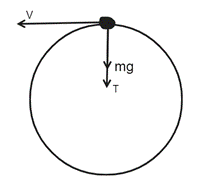The velocity of the object, v, is tangential to the circular path, pointing in the direction of the object’s circular motion. This is known as tangential velocity. Tangential velocity is the velocity of an object moving in a circular path in a direction tangent to the circle. It is perpendicular to the centripetal force and is constant for an object moving in a circular path at a constant speed.

T represents the tension force that pulls the object to the center, and mg is the force due to gravity, or the weight, that acts on the object. These two forces act towards the center of the object’s circular path, making them positive forces.

Conversely, if the object was at the bottom of its circular path, the tension force would still act towards the center, while the force due to gravity would point away from it, making the force negative. This is because gravity always pulls down on an object. The FBD of this situation would look like this: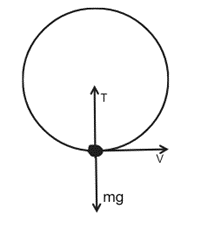The tension and gravitational forces are equal in magnitude (indicated by the arrow lengths) because the object hasn’t broken away from its circular path. Essentially, the string creates an equal and opposite tension force in reaction to gravity, keeping the object in circular motion.

#### Real Life Application: Roller Coaster

The FBDs provided can be translated in other areas of life as well. Case in point, roller coasters. Roller coasters are abundant with twists, turns, and most importantly, vertical loops. A vertical loop is a circular path that facilitates the application of forces on a roller coaster car.

First, the car enters the loop, experiencing the centripetal force that keeps it in a circular path. As the car approaches the top of the loop, it begins to slow down due to the gravitational force that acts on it.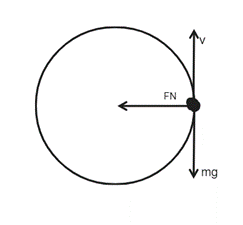Then, at the top of the loop, there is a temporary feeling of weightlessness that all riders experience. At this point, the car is moving at such a speed that a centripetal force greater than the force due to gravity is created, causing the car to accelerate upwards. Despite the normal force (FN)(1) and gravitational force pointing in the same direction, the car does not fall. The only way for the car to fall would be if it lost speed.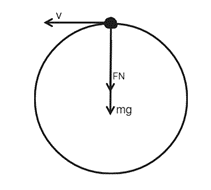After this, the car proceeds through the rest of the loop. The car accelerates downwards, adding to the downwards force of gravity and consequently makes the riders feel heavier once they’ve reached the bottom of the loop.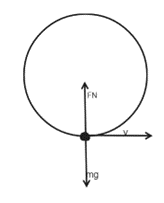#### Conclusion

Circular motion is a topic that requires a lot of conceptualization and real-world comprehension. By making connection between Newton’s laws of motion and circular motion along with understanding the relationship of certain variables in equations, real world scenarios can be seen through a physicists’ lens. Although aspects like friction and air resistance, aren’t present in this article, readers can acquire a broader perspective of life and how physics is applied to it.

1: Force normal, or the normal force, is a force perpendicular to the surface and is an example of Newton’s third law of motion that states every action has an equal but opposite reaction. Knowing this is essential to this real-world application because FN prevents objects from phasing through whatever surfaces they are on.